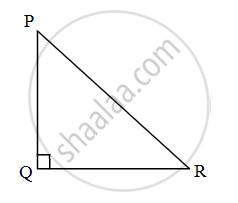# A ladder 10 m long reaches a window 8 m above the ground. Find the distance of the foot of the ladder from the base of wall. Complete the given activity. - Geometry

Sum

A ladder 10 m long reaches a window 8 m above the ground. Find the distance of the foot of the ladder from the base of wall. Complete the given activity.

Activity: As shown in figure supposePR is the length of ladder = 10 m

At P – window, At Q – base of wall, At R – foot of ladder

∴ PQ = 8 m

∴ QR = ?

In ∆PQR, m∠PQR = 90°

By Pythagoras Theorem,

∴ PQ2 + square = PR2    .....(I)

Here, PR = 10, PQ = square

From equation (I)

82 + QR2 = 102

QR2 = 102 – 82

QR2 = 100 – 64

QR2 = square

QR = 6

∴ The distance of foot of the ladder from the base of wall is 6 m.

#### Solution

As shown in figure suppose PR is the length of ladder = 10 m,

At P – window, At Q – base of wall, At R – foot of ladder

∴ PQ = 8 m

∴ QR = ?

In ∆PQR, m∠PQR = 90°

By Pythagoras Theorem,

∴ PQ2 + QR2 = PR2    .....(I)

Here, PR = 10, PQ = 8

From equation (I)

82 + QR2 = 102

QR2 = 102 – 82

QR2 = 100 – 64

QR2 = 36

QR = 6

∴ The distance of foot of the ladder from the base of wall is 6 m.

Concept: Similarity in Right Angled Triangles
Is there an error in this question or solution?

Share